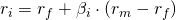# CAPM

The Capital Asset Pricing Model (CAPM) is based on the results we obtained from the capital allocation line and Markowitz’s portfolio selection. In particular, the CAPM assumes that all investors perform the same portfolio optimization we described in those sections.

If we make this assumption, as well as the following set of addition assumptions, we find some really useful results that we can use for portfolio construction and the valuation of individual securities.

If we assume that every investor

1. is small enough not to influence prices (no market power)
2. uses Markowitz portfolio selection approach
3. shares the same expectations with regard to expected returns, standard deviations and correlations
4. has the same investment horizon
5. relies on the same (free) information
6. can borrow and lend at the risk-free rate

then it means that all investors are sharing the same efficient frontier. That makes sense, as they are using the same inputs and use the same approach. Of course, investors can still have different combinations of the risk-free asset and the risky asset. So they are not necessarily holding the same overall portfolio. However, they are all holding an optimal risky portfolio that’s identical. In fact, all investors are holding the market portfolio.

The resulting capital allocation line is called the capital market line (CML).

## Security characteristic line (SCL)

The CAPM enables us to calculate the required return on a security using the risk free rate, the systematic risk of the security and the equity-risk premium. This required return can then be used to value equities, e.g. using a dividend discount model. In practice, securities with a beta higher than 1 are considered agressive securities, since they on average move more than the market. Securities with a beta lower than 1 are called defensive securities, since they move less than the market.## Summary

The capm, capital asset pricing model, outlines the required return on an asset in function of the risk free rate, the systematic risk component and the market premium.